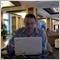# Highest high for an indicator81

I am trying to find out how to calculate the highest high and lowest low values for an indicator over a period in MQL4.

For example:

Using amibroker (similar sor metastock) the formula would be as below to calculate the highest high of the 30 day moving average over the last 10 periods.

Highest high = HHV(MA(Close,30),10 )

I have tried using ArrayMaximum() but this returns the position and not the value of the highest value.

Also Highest() does not work with indicators.

Any help will be much appreciated318

Hi...............................

Files:
hh_ll.mq4 3 kb5921476

Take a look at the dictionary :

int Highest( string symbol, int timeframe, int type, int count=WHOLE_ARRAY, int start=0)

Returns the shift of the maximum value over a specific number of periods depending on type.

Do not forget that this function return the index of the bar, not a value, so look at the sample :

Sample:

double val;

// calculating the highest value in the range from 5 element to 25 element

// indicator charts symbol and indicator charts time frame

val=High;81

Thanks for all the replies.

Michel,

I think the formula:

val=High;

Will return the highest high of the high price for that period but can not be used to return the highest high for an indicator like the moving average of the price.

This is because the series array identifier used is MODE_HIGH.

Please correct me if I am wrong.318

Files:592181

Ok I have found a way to calculate the highest high or lowest low for an indicator over a specified period.

If you wanted to find the highest high or lowest low for an indicator like the simple moving average you have to declare an array and fill a "for" loop with the calculated values for each bar of the period you are testing.

Then you have to use ArrayMaximum and ArrayMinimum to find the position of the high or low in the array and then find the value of that position using iHigh() and iLow().

//calculate the highest high of the moving average

int malookback=5; //4+1 - the number of bars to calc the value of the ma

int madailyPERIOD = 30; //moving average period

double dhhv,dllv;

for(int i = 0; i < malookback; i++)

{

//calculate the lowest low of the moving average

}220

i-HighLow Indicator

i-HighLow indicator draws the channel with borders correspond to highest high and lowest low values of the bar's shadows for the last N bars.

Indicators' parameters are:

extern int N = 20; - searching of highest/lowest bars for the last 20 bars

extern int N2 = 5; - drawing the borders with 5 points offset by price axis

Files:
hi_low1.gif 35 kb
hi_low2.gif 38 kb108

HH HL LH LL indicator

Hi all !

Do you know an indicator (or Expert advisor) that mark the HH/HL/LH/LL on the chart?

Thanks,

YanModerator
117540

I moved your post to this thread where you can find some coding decision and indicators.

Also you can look at those threads/posts: